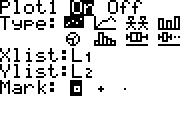# Steps for using the TI-73+ for finding models for data

(regression models)

Enter Data (1)

> press LIST and type ordered pairs in L1 and L2

(hint: to quickly clear an old list, go to the top and press CLEAR)

To look at a scatterplot

 > press 2nd PLOT and make sure that a plot (probably Plot1) is set properly for a scatterplot (On, scatterplot, x-list L1, y-list L2 as shown at the right) Plot2 and Plot3 should be OFF. > press ZOOM, 7:ZoomStat (which also sends you to GRAPH) (> press GRAPH anytime to go to the graph)To find the model (equation)

> press 2nd STAT, CALC

> press LinReg, QuadReg, or ExpReg... (for linear, quadratic, and exponential regression, respectively)

> press ENTER (assuming you used L1 and L2) (if you wish to use other lists, they must be specified))

Load the equation into the calculator for future use

> press Y=

(> CLEAR out the old equations--if you didn't do this initially)

> press 2nd VARS (for variables), Statistics, EQ, RegEQ (for regression equation)

To see your model graphed on top of the scatterplot

> press GRAPH (2)

To plug in an x-value into your model (3)

> press 2nd VARS (for variables), Y-Vars, Y1 (or whichever function you loaded it into), "(#)" where # is a number, ENTER

Footnotes

1. Before entering data, it is good practice to press Y= and CLEAR all existing equations.

2. TRACE works nicely at this point. steps x right and left. switches from the data to the model and back.

3. The other way to plug in an x-value(s) is to use the Table, using TBLSET and TABLE.

Jim Olsen, Western Illinois University